# JEE Main 2020 Question Paper with Solution (Jan 8th second shift)

## 8th January, Afternoon Session ( 2:30 pm to 5:30 pm)

As we all know JEE main 2020 exam is conducted by the National test agency through online mode. The second day of the exam was the 8th of January which is held in two shifts Morning and Afternoon. As the JEE main 2020 exam on 8th January successfully conducted, aspirants may be eager to know the type of questions and level of exam. Through this article, you will get JEE main 2020 question paper with solution (Jan 8th second shift). Aspirants who appeared for the JEE main 2020 exam can check the solution and check their performance here.

JEE Main 2020 exam - Chemistry ( 8th January second shift)

Q1) What are the two monomers of maltose that are formed on hydrolysis?

Solution

The two monomers of maltose are:

-D-glucose and -D-glucose

Q2) Which of the following is the correct order of the atomic radii of the elements C, O, F, Cl and Br?

Solution

The correct increasing order of the atomic radii of elements is given below:

F < O < C < Cl < Br

Atomic radii increase down a group and it decreases from left to right in a period.

Q3) Which of the following won't give Kjeldahl's test?

(a) C6H5NH2
(b) C6H5NO2
(c) NH2CONH2
(d) CH3CH2CN

Solution

Kjeldahl's method is not applicable to compounds containing nitrogen in nitro and azo compounds and nitrogen present in rings(pyridine) as nitrogen of these compounds does not convert to ammonium sulphate.

Therefore, Option(b) is correct.

Q4) Which of the following will show both frenkel and schottky defect?

1) AgBr

2) KBr

3) CaCl2

4) ZnS

Solution

Only AgBr can exhibit both Schottky and Frenkel defect.

Option 1 is correct

Q5) Arrange the different bonds according to their bond energies:

C-F, C-Cl, C-Br and C-I

Solution

The values of bond energies are given below:

C-F         -          485kJ/mol

C-Cl       -           328kJ/mol

C-Br       -           276kJ/mol

C-I         -            240kJ/mol

Thus, the correct order of bond energies is:

C-I > C-Br > C-Cl > C-F

Q6) Determine total number of neutrons in three isotopes of hydrogen.

(1) 1

(2) 2

(3) 3

(4) 4

Solution

Number of neutrons in Protium is 0.
Number of neutrons in Deuterium is 1.
Number of neutrons in Tritium is 2.

Thus, the correct answer is 0 + 1 + 2 = 3.

Q7) Determine Bohr's radius of Li2+ ion for n = 2. Given (Bohr's radius of H-atom = a0)

Solution

Option 2 is correct.

Q8) How many atoms lie in the same plane in the major product (C) ?

(Where A is the alkyne of lowest molecular mass)

Solution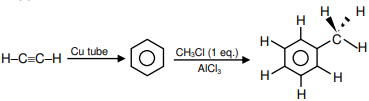Number of atoms in one plane = 13.

Q9) Complex [ML5] can exhibit trigonal bipyramidal and square pyramidal geometry. Determine the total number of 180º, 90º & 120º L-M-L bond angles.

SolutionThus, the correct answer is 20.

Q10) Given the following reaction sequence(1) Mg, Mg3N2
(2) Na, Na3N
(3) Mg, Mg(NO3)2
(4) Na, NaNO3

Solution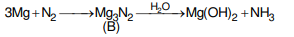Option 1 is correct

Q11) Correct order of magnetic moment (spin only) for the following complexes

(a) [Pd(PPh3)2Cl2]

(b) [Ni(CO)4]

(c) [Ni(CN)4]2–

(d) [Ni(H2O)6]2+

(1) a = b = c < d

(2) a < b < c < d

(3) a > b > c > d

(4) a = b > c > d

SolutionOption 1 is correct.

Q12)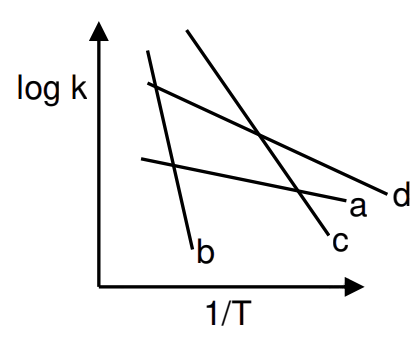Compare Ea (activation energy) for a, b, c and d.

(1) Eb > Ec > Ed > Ea

(2) Ea > Ed > Ec > Eb

(3) Ec > Eb > Ea > Ed

(4) Ed > Ea > Eb > Ec

Solution

Eb > Ec > Ed > Ea

Option 1 is correct.

Q13) Given:

Basicity of B is:

(1) 1

(2) 2

(3) 3

(4) 4

Solution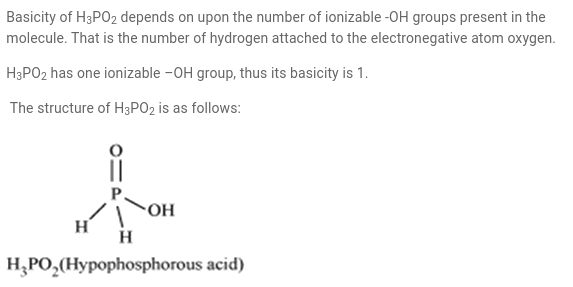Option 1 is correct.

Q14) Which reaction does not occurs in the blast furnace in the metallurgy of Fe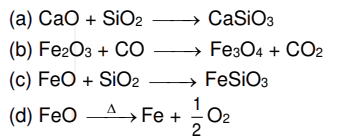(1) a & b

(2) a,b & c

(3) c & d

(4) a, b, c, d

Solution

Concept Based

Option 3 is correct.

Q15)  Correct order of radius of elements is:   C, O, F, Cl, Br
(1) Br > Cl > C > O > F

(2) Br < Cl < C < O < F

(3) Cl < C < O < F < Br

(4) C > F > O > Br > Cl

SolutionOption 1 is correct

Q16) Amongs the following which will show geometrical isomerism.

(a) [Ni(NH3)5Cl]+

(b) [Ni(NH3)4ClBr]

(c) [Ni(NH3)3Cl]+

(d) [Ni(NH3)2(NO2)2]

(1) b, d

(2) a, b

(3) a, b & c

(4) a, b, c & d

Solution

Ma4bc can show 2 G.I.
Ma2b2 can show 2 G.I.
(Square planar)

Option 1 is correct.

Q17) Assertion: pH of water increases on increasing temperature.
Reason:
is an exothermic process.
(1) Both assertion and reason are correct and reason is correct explanation of assertion.
(2) Both assertion and reason are correct and reason is not correct explanation of assertion.
(3) Assertion is true & reason is false.
(4) Both assertion and reason are incorrect.

Solution

Concept Based.

Option 4 is correct.

Q18)  Assertion: It has been found that for hydrogenation reaction the catalytic activity increases from group5 to group-11 metals with maximum activity being shown by groups 7-9 elements of the periodic table.
Reason: For 7-9 group elements adsorption rate is maximum.
(1) Both assertion and reason are correct and reason is correct explanation of assertion.
(2) Both assertion and reason are correct and reason is not correct explanation of assertion.
(3) Assertion is true & reason is false.
(4) Both are incorrect

Solution

Concept Based.

Option 1 is correct.

Q19) Given following reaction,

In the above reaction, 492 L of O2 is obtained at 1 atm & 300 K temperature. Determine mass of NaClO3 required (in kg). (R = 0.082 L atm mol–1 K–1)

Solution

moles of NaClO3 = moles of O2

Mass of NaClO3 = 20 x 106.5 = 2130g

Q20) Temperature of 4 moles of gas increases from 300 K to 500 K find 'Cv' if U = 5000 J.

Solution

U = nCvT

5000 = 4 × Cv (500 – 300)

Cv = 6.25 JK–1 mol–1

Thus, the correct answer is 6.25

Q21) Formation of Bakelite follows:

(a) Electrophilic substitution followed by condensation.
(b) Nucleophilic addition followed by dehydration.
(c) Electrophilic addition followed by dehydration.
(d) Hydration followed by condensation.

Solution

Formation of Bakelite follows electrophilic substitution reaction of phenol with formaldehyde followed by condensation.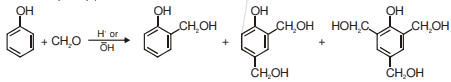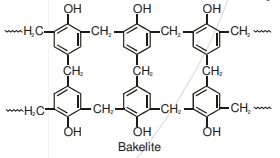Q22) A compound X that adds 2 hydrogen molecules on hydrogenation. The compound X also gives 3-oxohexanedioic acid on oxidative ozonolysis. The compound 'X' is:SolutionQ23) There are two compounds A and B of molecular formula C9H18O3. A has higher boiling point than B. What are the possible structures of A and B?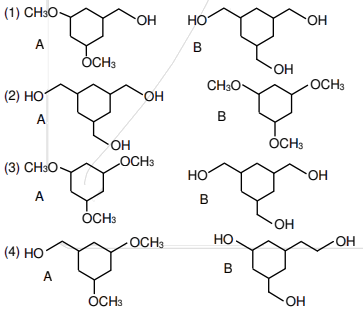Solution

In (A), extensive inter-molecular H-bonding is possible while in (B) there is no Inter-molecular H-bonding.

Therefore, Option(2) is correct.

Q24) Find the final major product of the following reactions: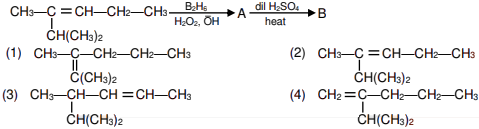SolutionTherefore, Option(1) is correct

Q25) The major product of the following reactions is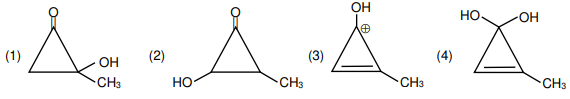Solution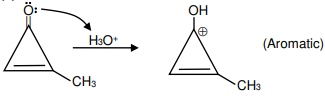Therefore, Option(3) is correct.

JEE Main 2020 exam - Mathematics ( 8th January second shift)

Q1. If a hyperbola has vertices (±6, 0) and P(10, 16) lies on it, then the equation of normal at P is

Solution:

Vertex is at (±6, 0)

a = 6

Let the hyperbola is

Putting point P(10, 16) on the hyperbola

Q2. How many 4 letter words can be formed with/without meaning using the word EXAMINATION?

Solution:

EXAMINATION       2N, 2A, 2I, E, X, M, T, O

Case I     All are different so

Case II    2 same and 2 different so

Case III    2 same and 2 same so  = 18

Total = 2454

Q3. In an A. P.  10th term is 1/20.and 20th term is 1/10.then find the sum of first  200th term

Solution:

Q4. The total number of observations is 20, the mean of the observation is 10 and the variance of the observation is 4  when taking incorrect reading 9 in place of 10. The correct variance is?

Solution:

Q5. Find the domain of the function  having range (1,3)

Solution:

If x lies (1,2) [x]=1

Domain is

If x lies [2,3) [x]=2

Domain is .

Q6. Let   is nonzero vector and

Solution:

Q7. Let the coefficient of and in the expansion of  is  and  then  is equal to

Solution:

Q8. Differential equation of , where b is a parameter, is

Solution:

So the Differential equation is

Q9. Image of (1, 2, 3) w.r.t a plane is then which of the following points lie on the plane

Solution:

P(1,2,3) and Q

Direction ratio of normal to the plane

we get

and Midpoint of PQ is

equation of plane x + y + z = 1

Q10.

Solution:

Using L'Hospital

Q11. Let P be the set of points (x, y) such that . Then area of region bounded by points in set P is

Solution:

Point of intersection of  is – 3, 1

Now,

Q12.

Solution:

Q13. Let the line y = mx intersects the curve  at P and tangent to  at P intersects x-axis at Q. If area (OPQ) = 4, find m (m > 0)

Solution: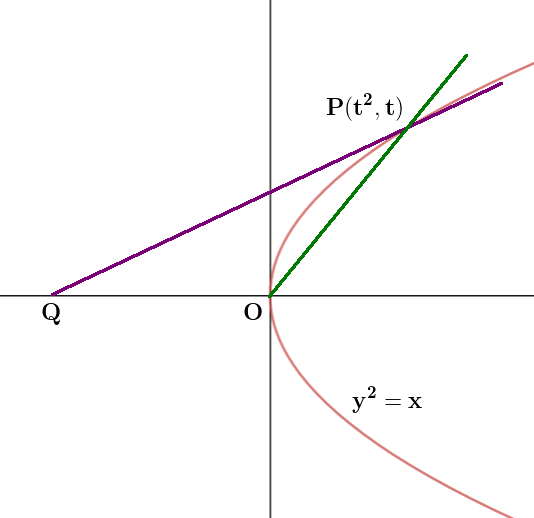Q14. Let  where  Then tan () is equal to

Solution:

Q15. If y = mx + c is a tangent to the circle  and also the perpendicular to the tangent to the circle  at  then

Solution:

The slope of the tangent to

so m = 1

y = x + c

now distance of (3, 0) from y = x + c is

Q16. Which of the following is a tautology

Solution:

Q17. Normal at (2, 2) to curve  L. Then the perpendicular distance from the origin to line L is

Solution:

(2, 2) satisfy x – y = 0

Normal

x + y = = 4 Hence

the perpendicular distance from the origin

Q18. Let

Solution:

Q19. Let  then value of is

Solution:

On comparing we get

Q20. x + 2y + 2z = 5, 2x + 3y + 5z = 8 and 4x + y + 6z = 10 for the system of equation check the correct option.

(1) Infinite solutions when = 8                   (2) Infinite solutions when  = 2

(3) no solutions when  = 8                         (4) no solutions when =2

Solution:

D = ( + 8) ( 2 – ) for  = 2

= 5[18 – 10] – 2 [48 – 50] + 2 (16 – 30]

= 40 + 4 – 28  0 No solutions for  = 2

Q21. Let  ,

If a and b are roots of quadratic equation then quadratic equation is ?

Solution:

= , b = 1 + 3 + 6 + ……= 101

a= (1+) (1+2+4+6.....200)

x2 – 102x + 101 = 0

Q22. Let f(x) is a three degree polynomial for which f ' (–1) = 0, f '' (1) = 0, f(–1) = 10, f(1) = 6 then local minima of f(x) exist at

Solution:

Let f (x) = ax3 + bx2 + cx + d

a = 1/4, d = 35/4, b = –3/4,  c =9/4

f(x) = a(x3 – 3x2 – 9x) + d

f ' (x) = 0  x = 3, –1

local minima exist at x = 3

Q23. Let A and B are two events such that  then

Solution:

Q24. Solution set of  contains

(1) singleton set

(2) two elements

(3) at least four elements

(4) infinite elements

Solution: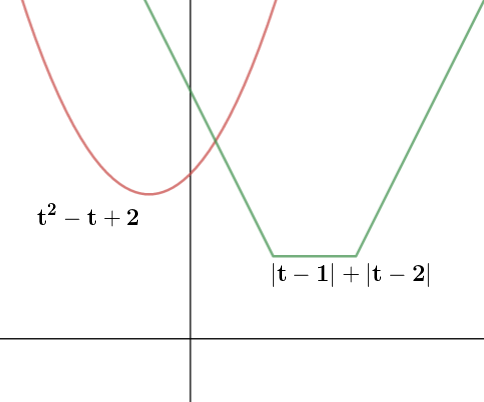singolton set

JEE Main 2020 exam - Physics ( 8th January second shift)

Q-1) The efficiency of a carnot engine is 1/10. If this carnot engine is used as a refrigerator and the work required by it is 10J. Find the refrigeration effect of the refrigerator.

Sol-

Q-2 Find magnetic field at O.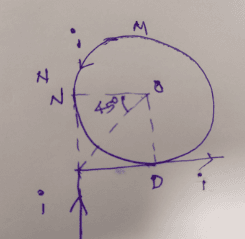Solution-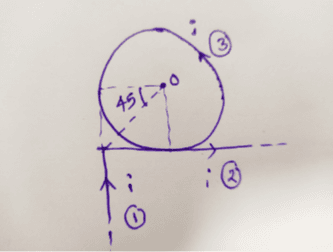Magnitude of net magnetic field at O is

The direction of magnetic field due to first conductor ( in to the plane) is opposite to that of other two conductors (out of the plane).

Q 3- Position of particle as a function of time is given as . Choose correct statement about where  are velocity and acceleration of particle at time t.

Options-

(1)  is perpendicular to  and  is towards origin

(2) are perpendicular to

(3)  is parallel to   and   parallel to

(4)  is perpendicular to and is away from origin.

Sol-

implies a is anti parellel to r

Implies v is perpandicular to r

So option 1 is correct

Q 4- Two uniformly charged solid spheres are such that E1 is electric field at surface of 1st sphere due to itself. E2 is electric field at surface of 2nd sphere due to itself. r1, r2 are radius of 1st and 2nd sphere respectively. If  then ratio of potential at the surface of spheres 1st and 2nd due to their self charges is:

Options:

sol-

Given

Q-5

Output at terminal Y of given logic circuit is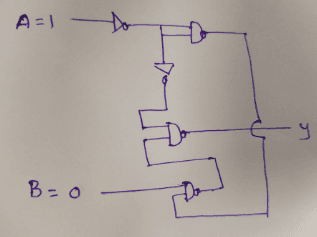Options-

1) 0    2) 1    3) oscillating between o and 1    4) not determined

Sol-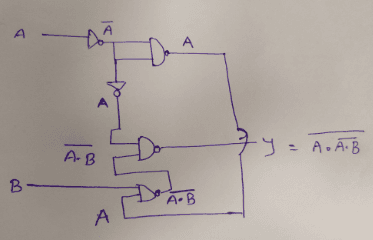Q 6- Velocity of a wave in a wire is v when tension in it is 2.06 × 104 N. Find value of tension in wire when velocity of wave become v/2.

Options:

(1) 5.15 × 103 N (2) 8.24 × 104 N (3) 6 × 104 (4) 5.15 × 104 N

Sol-

Velocity

Q 7- n mole of He and 2n mole of O2 is mixed in a container. Then   will be

Options:

Sol-

For mono atomic gas (He)

For diatomic gas (O2)

Q-8 A uniform solid sphere of radius R has a cavity of radius 1m cut from it if centre of mass of the system lies at the periphery of the cavity then

Options

Sol-

Shift in centre of mass = 2-R

Q-9

A solid sphere of mass m= 500gm is rolling without slipping on a horizontal surface. Find kinetic energy of a sphere if velocity of centre of mass is 5 cm/sec.

Option:

Sol-

The kinetic energy of sphere is kinetic energy of translation+ kinetic energy of rotation

Where K is the radius of gyration

Q-10

Two liquid columns of same height 5m and densities  and  are filled in a container of uniform cross sectional area. Then ratio of force exerted by the liquid on upper half of the wall to lower half of the wall.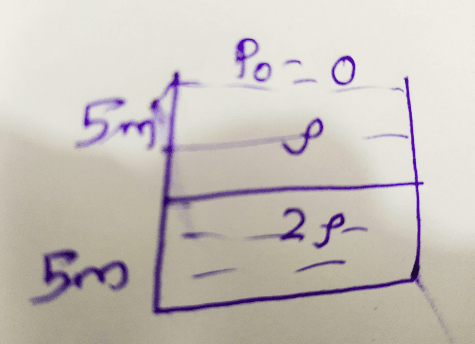Sol-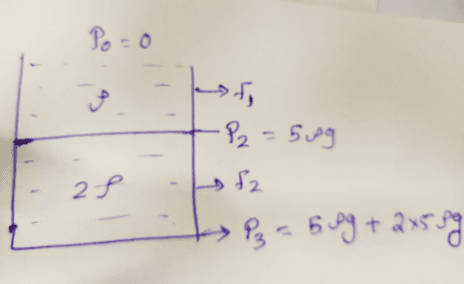Q-11

Two square plates of side 'a' are arranged as shown in the figure. The minimum separation between plates is 'd' and one of the plate is inclined at small angle  with plane parallel to another plate. The capacitance of capacitor is (given  is very small)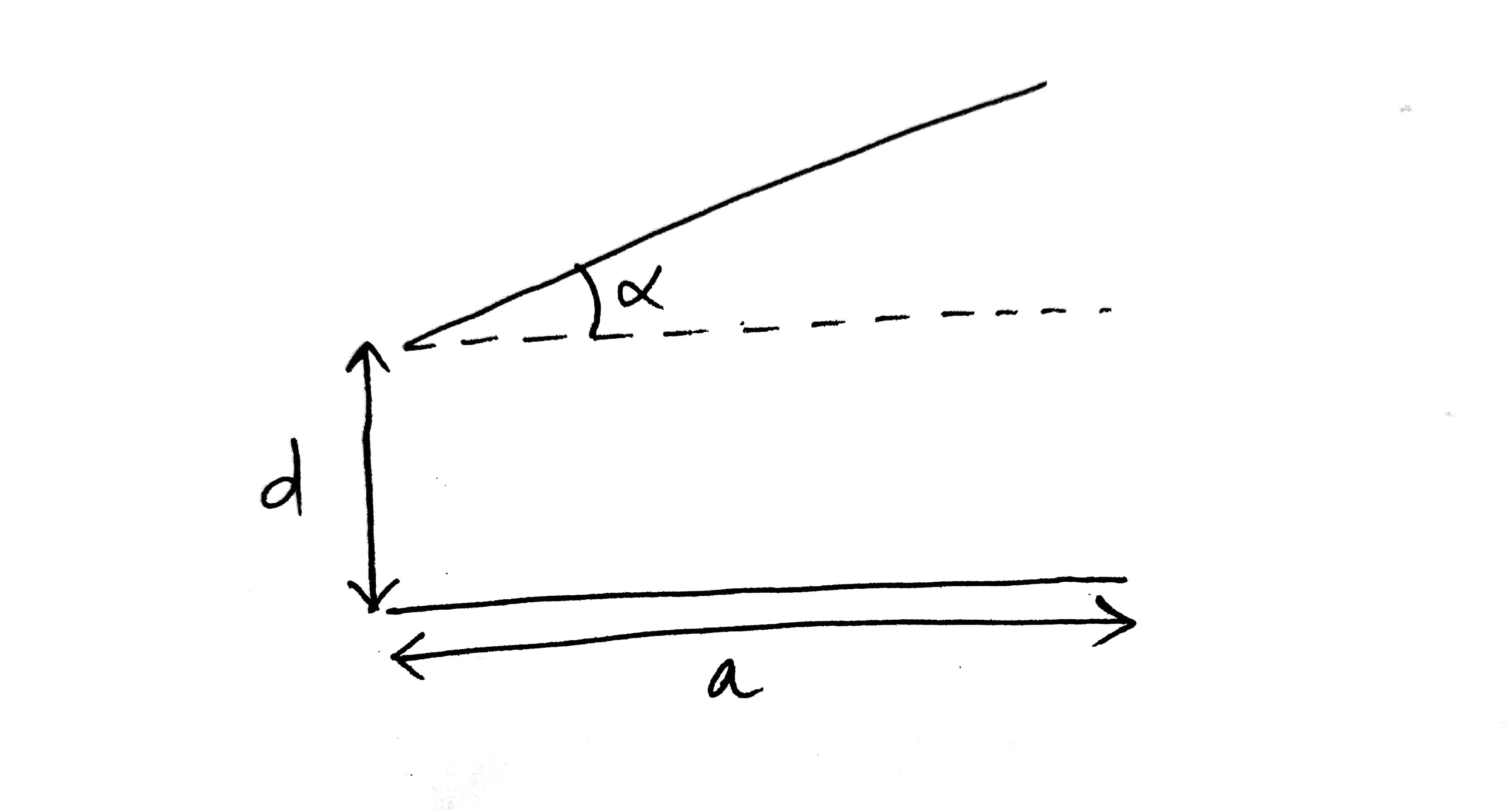Solution-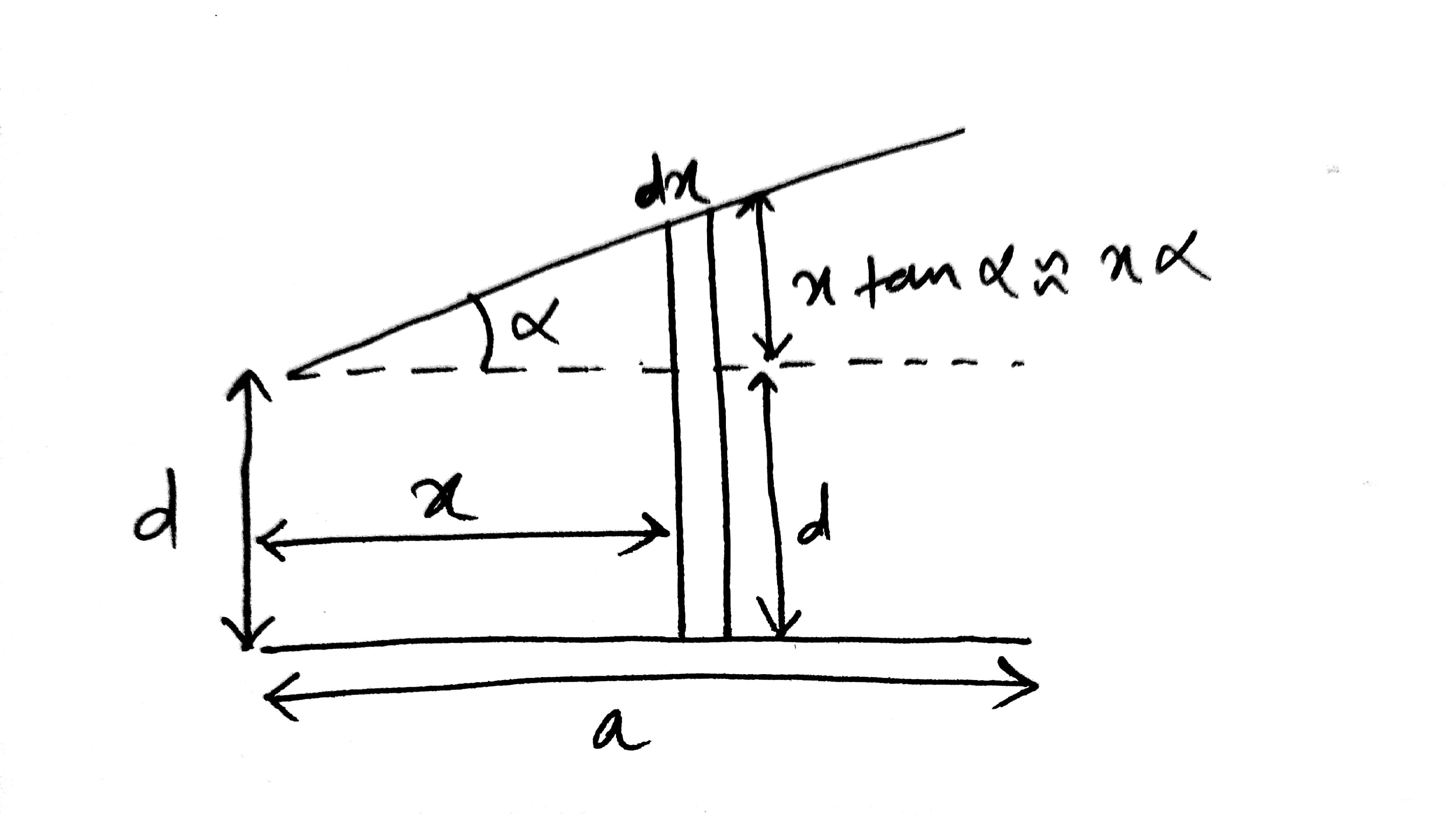Take an elemental strip of length dx at a distance x from one end

So the capacitance of an elemental strip is

So integrating this will give total capacitance=

(Using )

Q-12

In YDSE path difference at a point on screen is   . Find ratio of intensity at this point with maximum intensity

Solution-

Intensity is given by

Where IO=maximum intensity

and  using the Relation

we get

Q-13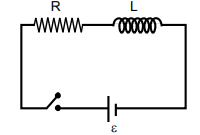Solution-

As current at any time t is given as

So

So integrating this will give total charge

Q-14

A particle is dropped from height h = 100 m, from surface of a planet. If in last (1/2) sec of its journey it covers 19 m. Then value of acceleration due to gravity that planet is :

Solution-

Let total time taken by particle is t

So distance covered by particle in t sec=

similarly  distance covered by partical in  sec=

So  distance covered by partical in last (1/2) sec of its journey=

So

and

from equation (1) and (2)

we get

putting t=5 we get g = 8 m/s2

Q-15

A charged particle of mass m and charge q is released from rest in a uniform electric field. Its graph between velocity (v) and distance traveled (x) will be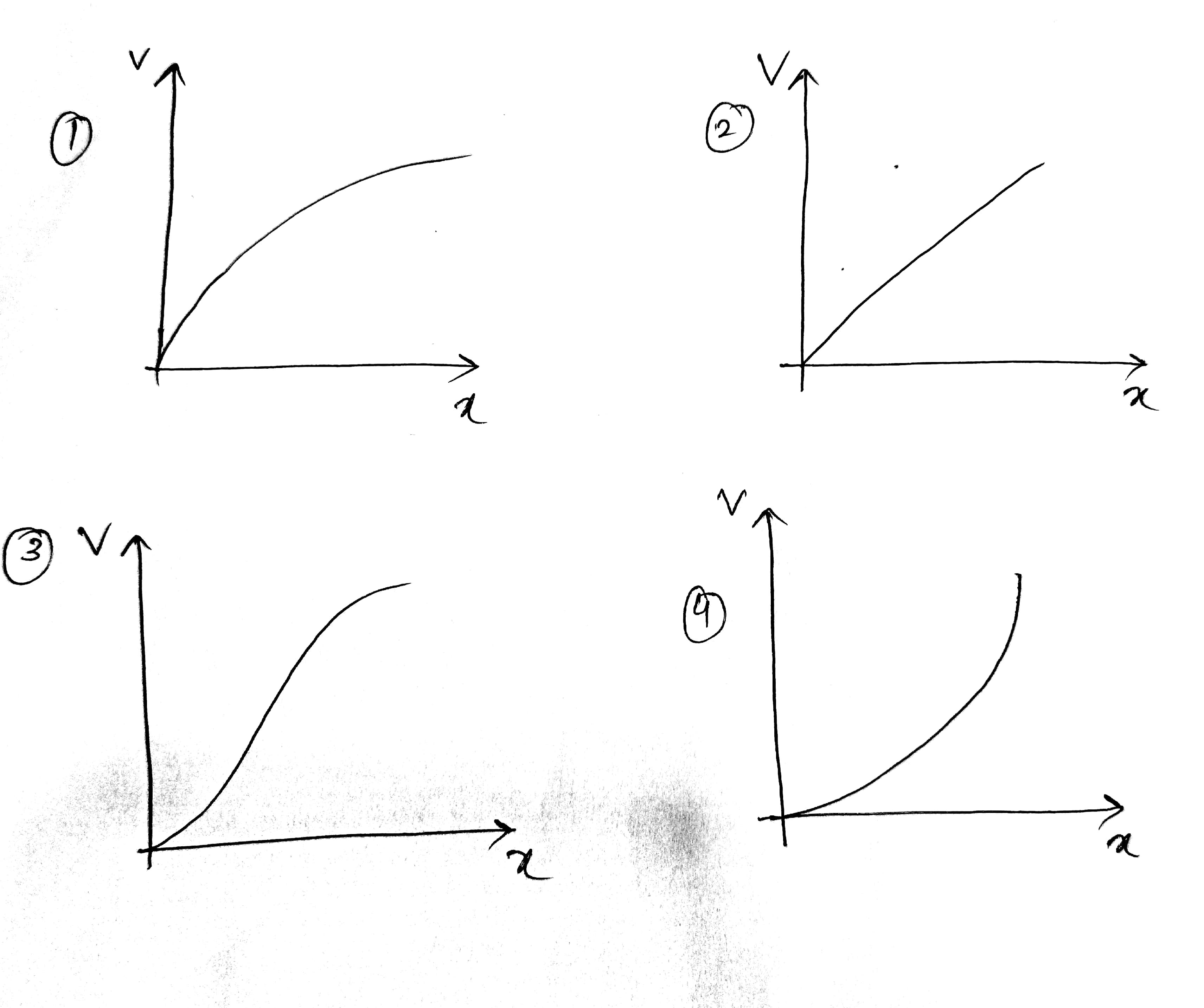Solution:-

So graph would be option 1

Q-16 An object is moving away from concave mirror of focal length f starting from focus. The distance of an object from pole of mirror is x. The correct graph of magnitude of magnification(m) verses distance x is: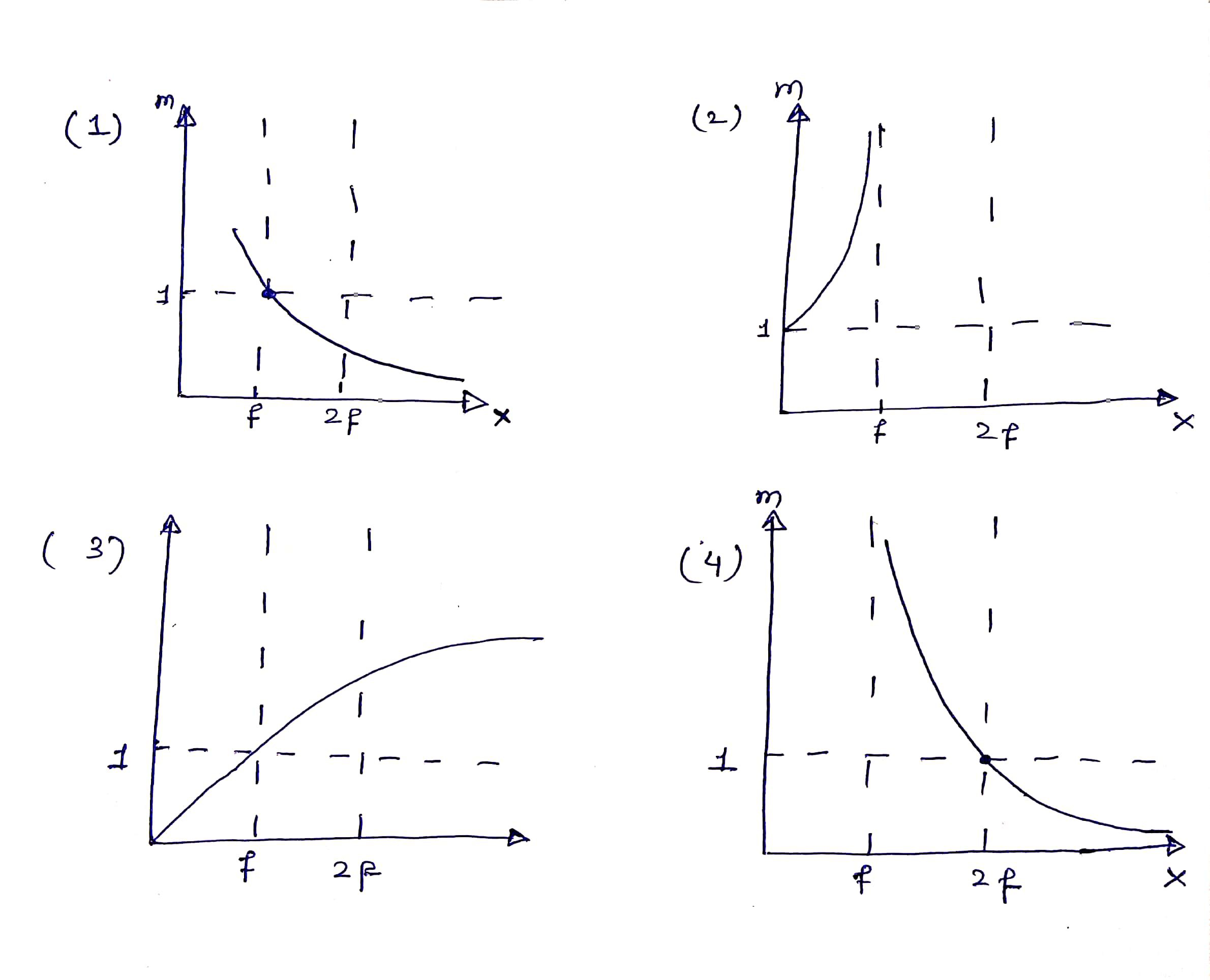Solution -

In this question we can use the elimination method by looking toward the graph.

As we know that the

And by using the mirror formula. we can write that the -

Now by using this equation, we can start eliminating the option. As we can see that the at u=f, the m become infinite. So the option (1) and (3) is incorrect. If we u = 2f, then the m =1.

So the option (3) is also wrong.

So the answer is option (4)

Q-17

In full scale deflection current in galvanometer of 100  resistance is 1 mA. Resistance required in series to convert it into voltmeter of range 10 V.

(1) 0.99 K                                                                                  (2) 9.9 K

(3) 9.8 K                                                                                     (4) 10 K

Soultion -

Q-18

There are two identical particles A and B. One is projected vertically upward with speed from ground and other is dropped from height h along the same vertical line. Collision between them is perfectly inelastic. Find time taken by them to reach the ground after collision in terms of     .

Solution -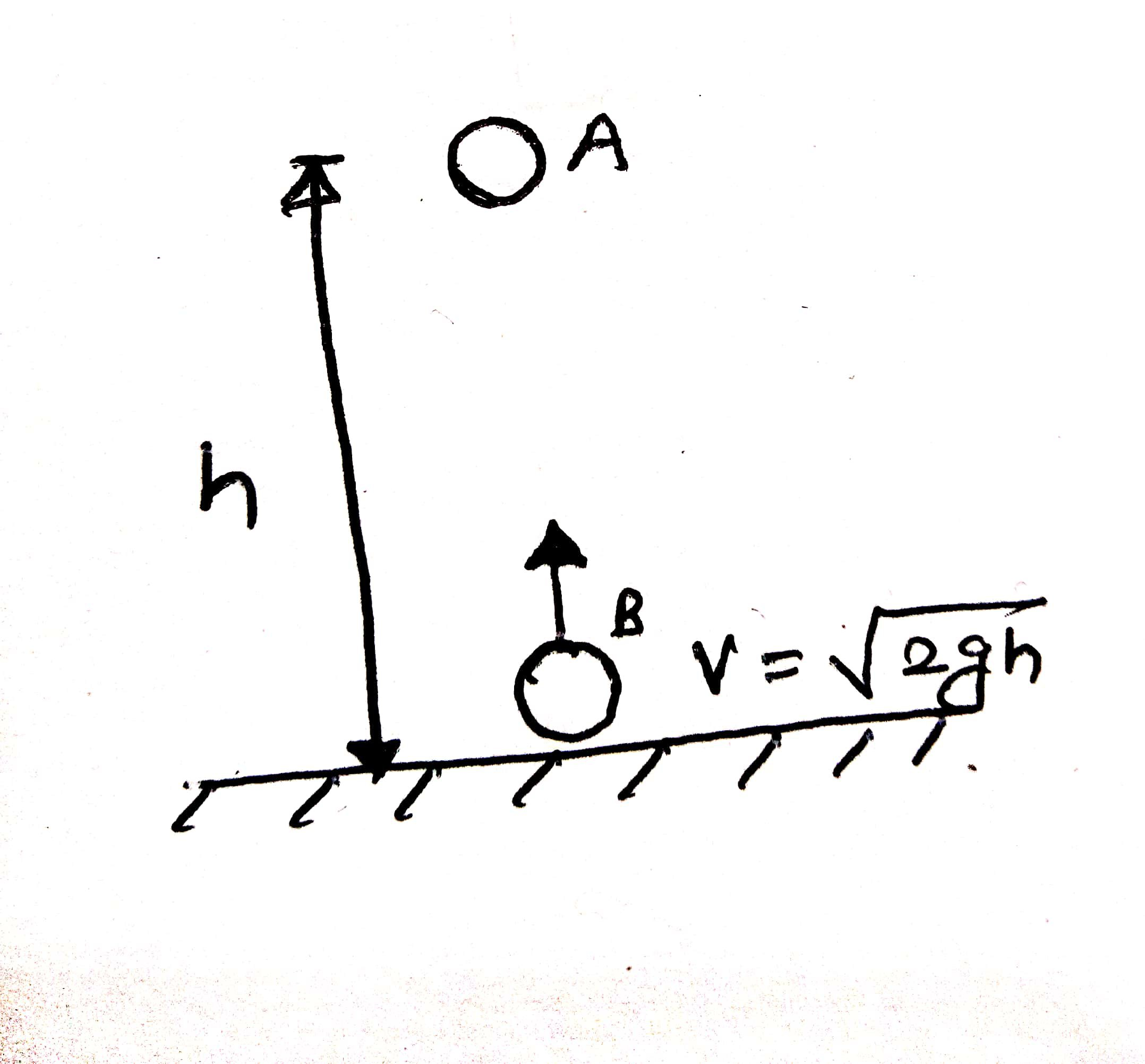time for collision -

After t1 -

and

at the time of collision

and height from ground

so time

So the option (1) is correct

Q-19

Length of a simple pendulum is 25.0 cm and time of 40 oscillation is 50 sec. If resolution of stop watch is 1 sec then accuracy is g is (in %)

(1) 2.4                          (2) 3.4                                (3) 4.4                                          (4) 5.4

Solution-

Note - We are adding the error just for calculating maximum possible error.

Q-20

Solution:-

We know v=u+at and here

So, magnitude of velocity:-

Q-21

An asteroid of mass is approaching with a velocity when it is at distance of  from the centre of earth (where R is radius of earth). When it reaches at the surface of Earth, its velocity is (Nearest Integer) in km/s.

Solution:-

We will apply energy conservation here:-

Q-22

In H-spectrum wavelength of 1% line of Balmer series is . Find out wavelength of 2nd line of same series in nm.

Solution:-

Q-23

Solution:-

We know that:-  and here s is same

Q-24

Solution:-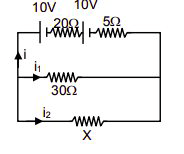Q-25

Solution:-